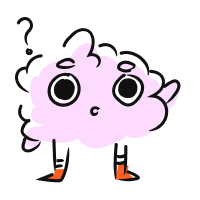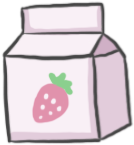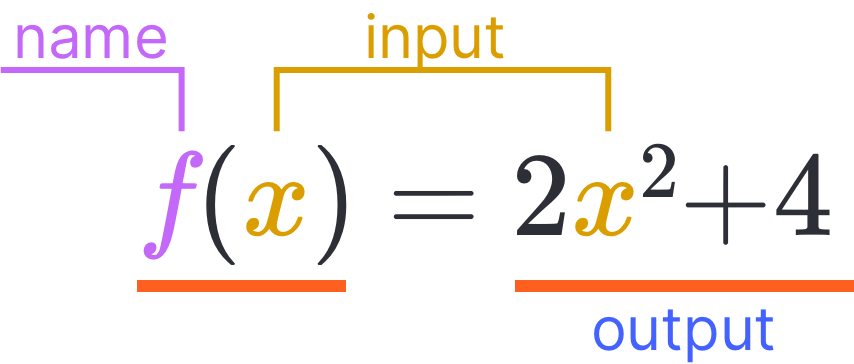STUDENT
TEACHER

Function Calculator

STUDENT
TEACHER

Function Calculator

# Functions

CALCULATOR

## Function Calculator

Function:
Evaluate:We have some questions for you! Help us out through this
INTRO
A function defines the relationship between an input and an output.

Functions operate a lot like vending machines. When we enter a code into a vending machine, we get a specific drink. When we enter an input into a function, we get an output. Try it out!

Function Vending Machine (drinks)A1A2A3B1B2B3C1

A
B
C
1
2
3
In order for the relationship to be considered a function, each input can only have one output. However, multiple inputs can have the same output.

In our vending machine above, this is like how each code gets us one specific drink (B1 always gives us water), but there can be multiple codes that can get us the same drink (to get water, we can input B1, B2, or B3).

### Parts of a FunctionThe function, named , takes some number as an input, squares it, multiplies by 2, and then adds 4 to produce its output.
Check out our
Calculator
or explore our
Lesson
and
Practice

KEY STEPS

## How to Evaluate a Function

### Step 1. Plug in the input.

Plug in the input wherever the variable shows up in the function.
Imagine this as entering the code for a drink we want from a vending machine.

### Step 2. Calculate the output.

Use order of operations (PEMDAS) to calculate the output.
Imagine this as the vending machine processing the code to dispense the drink.
LESSON
Evaluating Functions
PRACTICE
Evaluating Functions
CONCLUSION
Leave Feedback